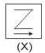>  Test: BITSAT Mock Test- 5

# Test: BITSAT Mock Test- 5

Test Description

## 150 Questions MCQ Test BITSAT Mock Tests Series & Past Year Papers | Test: BITSAT Mock Test- 5

 1 Crore+ students have signed up on EduRev. Have you?
Test: BITSAT Mock Test- 5 - Question 1

### In a purely resistive A.C. circuit, the current

Test: BITSAT Mock Test- 5 - Question 2

### A radioactive substance has a half-life of 1 year. The fraction of this material, that would remain after 5 years will be

Test: BITSAT Mock Test- 5 - Question 3

### In Bohr's model, the atomic radius of the first orbit is r₀ then the radius of the third orbit is

Test: BITSAT Mock Test- 5 - Question 4
Two rods of same material and length have their electric resistance in ratio 1:2. When both rods are dipped in water, the correct statement will be
Test: BITSAT Mock Test- 5 - Question 5

An electric dipole has the magnitude of its charge as q, and its dipole moment is p. It is placed in a uniform electric field E. If its dipole moment is along the direction of the field, then force acting on it and its potential energy are respectively

Detailed Solution for Test: BITSAT Mock Test- 5 - Question 5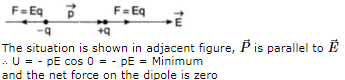Test: BITSAT Mock Test- 5 - Question 6

A body of mass 1g and carrying a charge 10−8 C passes from two points P and Q. P and Q are at electric potentials 600V and 0V respectively. The velocity of the body at Q is 20 cms−1 . Its velocity in ms−1 at P is

Test: BITSAT Mock Test- 5 - Question 7
The resistance of an ammeter is 13$Ω$ and its scale is graduated for a current upto 100 A. After an additional shunt has been connected to this ammeter it becomes possible to measure currents upto 750 A by this meter. The value of shunt resistance is
Test: BITSAT Mock Test- 5 - Question 8
A metal plate gets heated, when cathode rays against it, due to
Test: BITSAT Mock Test- 5 - Question 9
During X-ray formation if voltage is increased
Test: BITSAT Mock Test- 5 - Question 10
A capacitor of capacity C has reactance X. If capacitance and frequency become double, then reactance will be
Test: BITSAT Mock Test- 5 - Question 11

A car having a mass of 1000 kg is moving at a speed of 30 metres/sec. Brakes are applied to bring the car to rest. If the frictional force between the tyres and the road surface is 5000 newtons, the car will come to rest in

Detailed Solution for Test: BITSAT Mock Test- 5 - Question 11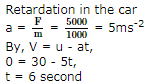Test: BITSAT Mock Test- 5 - Question 12
Time period of revolution of a satellite around a planet of radius R is T. Period of revolution around another planet, whose radius is 3R but having same density is
Test: BITSAT Mock Test- 5 - Question 13

A body cools from 60oC to 50oC in 10 minutes. If the room temperature is 25oC and assuming Newton's law of cooling to hold good, the temperature of the body at the end of the next 10 minutes will be

Test: BITSAT Mock Test- 5 - Question 14
Kinetic theory of gases was put forward by
Test: BITSAT Mock Test- 5 - Question 15

A sphere of radius r is falling in a viscous fluid. The terminal velocity attained by the falling sphere will be proportional to

Test: BITSAT Mock Test- 5 - Question 16
A uniform plank of Young's modulus Y, is moved over a smooth horizontal surface by a constant horizontal force F. The area of cross-section of plank is A. The compressive strain on the plank in the direction of the force is
Test: BITSAT Mock Test- 5 - Question 17
Raindrops are falling from a certain height. Assume all raindrops are spherical and have same drag coefficient. The impact speed of large raindrops compared to that of small raindrops is
Test: BITSAT Mock Test- 5 - Question 18
In a vibration magnetometer, the time-period of magnets when the identical poles are in the same directon is 3 s. When the polarity of weaker magnet is reversed, the combination makes 15 oscillations per minute. The ratio of magnetic moments of the magnets is
Test: BITSAT Mock Test- 5 - Question 19

An aeroplane is moving with horizontal velocity u at height h. The velocity of a packet dropped from it on the earth's surface will be (g is acceleration due to gravity)

Test: BITSAT Mock Test- 5 - Question 20
In the moving coil galvanometer the deflection is proportional to
Test: BITSAT Mock Test- 5 - Question 21

If a cycle wheel of radius 4 m completes one revolution in two seconds. Then acceleration of the cycle will be

Test: BITSAT Mock Test- 5 - Question 22
A particle covers 50 m distance when projected with an initial speed. On the same surface it will cover a distance, when projected with double the initial speed
Test: BITSAT Mock Test- 5 - Question 23
Two springs of the same material and same length have their tensions in the ratio 4:1 and redil in the ratio 2:1. The ratio of their fundamental frequencies is
Test: BITSAT Mock Test- 5 - Question 24
The magnifying power of a telescope is m. If focal length of its eye lens is halved, the magnifying power will become
Test: BITSAT Mock Test- 5 - Question 25
If the force on a rocket moving with a velocity of 300 m/s is 210 N. then the rate of combustion of the fuel is
Test: BITSAT Mock Test- 5 - Question 26

If a simple harmonic motion is represented by d2x/dt2 + αx = 0, its time period is

Test: BITSAT Mock Test- 5 - Question 27

The angle of minimum deviation for an incident light ray on an equilateral prism is equal to its refracting angle. The refractive index of its material is

Test: BITSAT Mock Test- 5 - Question 28

Two rings of the same radius and mass are placed such that their centres are at a common point and their planes are perpendicular to each other. The moment of inertia of the system about an axis passing through the centre and perpendicular to the plane of one of the rings is (mass of the ring = m and radius= r)

Test: BITSAT Mock Test- 5 - Question 29
A ring and a disc have the same mass and radius. The ratio of their moment of inertia about their axis is
Test: BITSAT Mock Test- 5 - Question 30
Masses 8, 2, 4, 2 kg are placed at the corners A, B, C, D respectively of a square ABCD of diagonal 80 cm. The distance of centre of mass from A will be
Test: BITSAT Mock Test- 5 - Question 31
If a high power heater is connected to electric mains, then the bulbs in the house become dim, because there is a
Test: BITSAT Mock Test- 5 - Question 32

A liquid of mass m and specific heat S is heated to a temperature T. Another liquid of mass m/2 and specific heat 2S is heated to a temperature 2T. If these two liquids are mixed, the resultant temperature of the mixture

Test: BITSAT Mock Test- 5 - Question 33
A process in which volume remains constant is called
Test: BITSAT Mock Test- 5 - Question 34

Which of the following pairs of physical quantities has the same dimensions

Test: BITSAT Mock Test- 5 - Question 35

A parallel monochromatic beam of light is incident normally on a narrow slit. A diffraction pattern is formed on a screen placed perpendicular to the direction of the incident beam. At the first minimum of the diffraction pattern, the phase difference between the rays coming from the two edges of slit is

Test: BITSAT Mock Test- 5 - Question 36
The superposition principle applies to
Test: BITSAT Mock Test- 5 - Question 37
The number of beats produced per second by the vibrations x₁ = a sin 320 π t and x₂ = a sin 326 π t is
Test: BITSAT Mock Test- 5 - Question 38
The momentum of a body is increased by 100% then its KE increases by
Test: BITSAT Mock Test- 5 - Question 39

A toy gun uses a spring of force constant k. When charged before being triggered in the upward direction, the spring is compressed by a distance x. If mass of the shot is m, then on being triggered it will go upto a height of

Test: BITSAT Mock Test- 5 - Question 40

Length of a string tied to two rigid supports is 40 cm. Maximum length (wavelength in cm) of a stationary wave produced on it is

Detailed Solution for Test: BITSAT Mock Test- 5 - Question 40

This will happen for fundamental mode of vibration as shown in the figure
Here, λ /2 = 40
∴ λ = 80 cm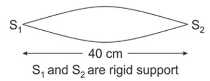Test: BITSAT Mock Test- 5 - Question 41
Epichlorohydrin is
Test: BITSAT Mock Test- 5 - Question 42
Cannizzaro's reaction is not given by
Test: BITSAT Mock Test- 5 - Question 43

Which one of the following statements is not correct

Test: BITSAT Mock Test- 5 - Question 44
The nitride ion in lithium nitrate is composed of
Test: BITSAT Mock Test- 5 - Question 45

How many atoms are contained in one mole of sucrose (C12H22O11)?

Detailed Solution for Test: BITSAT Mock Test- 5 - Question 45

C12H22O11 contains a total of 45 atoms ≅ 45 * 6.02 * 1023 atoms/mole

Test: BITSAT Mock Test- 5 - Question 46
0.126 g of acid required 20 mL of 0.1 N NaOH for complete neutralisation. The equivalent mass of an acid is
Test: BITSAT Mock Test- 5 - Question 47
Which of the following does not show electromeric effect?
Test: BITSAT Mock Test- 5 - Question 48
IUPAC name of CH₃-O-C₂H₅ is
Test: BITSAT Mock Test- 5 - Question 49
Night blindness is caused by the deficiency of
Test: BITSAT Mock Test- 5 - Question 50
A sugar, that is not a disaccharide, among the following is
Test: BITSAT Mock Test- 5 - Question 51
The acid showing salt like character in aqueous solutions is
Test: BITSAT Mock Test- 5 - Question 52
Tetrahedral nature of bonding in Carbon atom was first shown by
Test: BITSAT Mock Test- 5 - Question 53

What is the entropy change (in JK⁻1 mol⁻1) When one mol of ice is converted into water at 0oC. The enthalpy change for the conversion of ice to liquid water is 6.0 KJ mol⁻1 at )0oC.

Test: BITSAT Mock Test- 5 - Question 54

A Schematic plot of ln K (eq) versus inverse of temperature for a reaction is shown below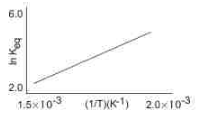The reaction must be

Test: BITSAT Mock Test- 5 - Question 55

The time taken for 90% of a first order reaction to complete is approximately

Test: BITSAT Mock Test- 5 - Question 56
Which of the following has largest radius
Detailed Solution for Test: BITSAT Mock Test- 5 - Question 56 As the number of electrons in an atom decreases, the effective nuclear charge or attractive force on the outer electrons increases whereas if the number of electrons increases, the effective nuclear charge decreases. Thus, the cation with highest charge always possess smallest ionic size and anion with highest charge always possess largest ionic size.
Test: BITSAT Mock Test- 5 - Question 57
The intensive property among these quantities is
Test: BITSAT Mock Test- 5 - Question 58
The rotenone is
Test: BITSAT Mock Test- 5 - Question 59
Potassium dichromate is obtained from which of the following ore?
Test: BITSAT Mock Test- 5 - Question 60
In the silver plating of copper, K[Ag(CN)₂] is used insead of AgNO₃. The reason is
Test: BITSAT Mock Test- 5 - Question 61
Super-conductors are derived from the compounds of
Test: BITSAT Mock Test- 5 - Question 62
The current in a given wire is 1.8 A. A charge passed through the wire in 1.36 minutes is
Test: BITSAT Mock Test- 5 - Question 63
When toluene is treated with KMnO₄,what is produced ?
Test: BITSAT Mock Test- 5 - Question 64

The compound which reacts with HBr obeying Markownikeff's rule is

Test: BITSAT Mock Test- 5 - Question 65

Heavy water is

Test: BITSAT Mock Test- 5 - Question 66
If H₂S is passed in ZnSO₄ containing HCl,Zinc sulphide is not precipitated because Ksp of
Test: BITSAT Mock Test- 5 - Question 67
A complete hydrolysis of cellulose gives
Test: BITSAT Mock Test- 5 - Question 68
Nitrogen dioxide
Test: BITSAT Mock Test- 5 - Question 69

The hybridized state of N in R₂NH is

Test: BITSAT Mock Test- 5 - Question 70
If chloroform is left open in air in presence of sun rays
Test: BITSAT Mock Test- 5 - Question 71
Antidote for carbon monoxide poisoning is
Test: BITSAT Mock Test- 5 - Question 72

Which of the following statements is false?

Test: BITSAT Mock Test- 5 - Question 73
N₂ and CO are
Test: BITSAT Mock Test- 5 - Question 74
NaHCO₃ is prepared by
Test: BITSAT Mock Test- 5 - Question 75
The fibre obtained by the condensation of hexamethylene diamine and adipic acid is
Test: BITSAT Mock Test- 5 - Question 76
Electrochemical process (electrolysis of fused salt) is employed to extract
Test: BITSAT Mock Test- 5 - Question 77

The lowering in vapour pressure, caused by the addition of 100 g of surcose (molecular weight = 342) in 1000 g of water, if the vapour pressure of pure water at 25oC is 23.8 mm of Hg, is

Test: BITSAT Mock Test- 5 - Question 78

How many Cd2⁺ ions surround each fluoride ion in cadmium fluoride crystal which adopts fluorite type structure?

Test: BITSAT Mock Test- 5 - Question 79
Liquid benzene burns in oxygen according to following equation. 2 C₆H₆(l) + 15 O₂(g) → 12 CO₂(g) + 6H₂O(g) How many litres of oxygen are required for complete combustion of 39 gram of liquid benzene ?
Test: BITSAT Mock Test- 5 - Question 80
Blood may be purified by
Test: BITSAT Mock Test- 5 - Question 81

the area of the curve x2 + y2= 2 ax is

Test: BITSAT Mock Test- 5 - Question 82
The positive integer just greater than (1 + .0001)10000 is
Test: BITSAT Mock Test- 5 - Question 83

Circles x2+y2-2x-y=0 and x2+y2-8y-4=0

Test: BITSAT Mock Test- 5 - Question 84

The abcissae of two points A, B are roots of x2 + 4x - 45 = 0. The centre of the circle for which AB are diameter is

Test: BITSAT Mock Test- 5 - Question 85

(1 − ω + ω 2)6 + (1 − ω2 + ω)6 =

Test: BITSAT Mock Test- 5 - Question 86

The solution of differential equation (dy/dx)+sin2y=0 is

Test: BITSAT Mock Test- 5 - Question 87

The number of solutions of y'=y+1/x-1, y(1)=2 is

Test: BITSAT Mock Test- 5 - Question 88

The solution of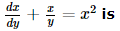Test: BITSAT Mock Test- 5 - Question 89

If f (x) = x − x2 + x3 − x4 + … ∞ , | x | < 1, then f ′ x =

Test: BITSAT Mock Test- 5 - Question 90

If cos(x+y)=y sinx, (dy/dx)=

Detailed Solution for Test: BITSAT Mock Test- 5 - Question 90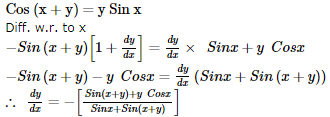Test: BITSAT Mock Test- 5 - Question 91
1/(1.2) - 1/(2.3) + 1/(3.4) - 1/(4.5) + ....... is
Test: BITSAT Mock Test- 5 - Question 92

The angle of elevation of the top of an unfinished tower at a point distant 120 m from its base is 45o. If the elevation of the top at the same point is to be 60o, the tower must be raised to a hight.

Test: BITSAT Mock Test- 5 - Question 93
If e and e1 are the eccentricities of the hyperbola xy = c2, x2 - y2 = c2 then ${e}^{2}+{e}_{1}^{2}$ is equal to
Test: BITSAT Mock Test- 5 - Question 94
The area of the region bounded by the lines x = 0, x = π/2 and f (x) = sin x, g (x) = cos x is
Test: BITSAT Mock Test- 5 - Question 95

The area between (x2/a2) + (y2/b2) = 1 and the straight line (x/a) + (y/b) = 1 is

Test: BITSAT Mock Test- 5 - Question 96

The value of 4tan⁻1(1/5)- tan⁻1(1/239) is

Test: BITSAT Mock Test- 5 - Question 97

If a matrix A is such that 3A3 + 2A2 + 5A + I = 0, then its inverse is

Test: BITSAT Mock Test- 5 - Question 98

log x2 is equivalent to

Test: BITSAT Mock Test- 5 - Question 99

1 • 2 + 2 • 3 + 3 • 4 + … n terms =

Test: BITSAT Mock Test- 5 - Question 100

If minimum value of f(x) = x2 + 2bx + 2c2 is greater than maximum value of g(x) = - x2 - 2cx + b2 then for x is real

Test: BITSAT Mock Test- 5 - Question 101
If the traces of the matrices A and B are 20 and -8, then the trace of (A + B)=
Test: BITSAT Mock Test- 5 - Question 102

The angle between pair of lines 2x2+5xy+2y2+3x+3y+1=0 is

Test: BITSAT Mock Test- 5 - Question 103
Imaginary part of 1/1+cos θ -i sin θ is
Test: BITSAT Mock Test- 5 - Question 104

The equation of the parabola with focus (3,0) and the directrix x+3=0 is

Test: BITSAT Mock Test- 5 - Question 105

A parallelogram is cut by two sets of m lines parallel to the sides, the number of parallelogram thus formed is

Test: BITSAT Mock Test- 5 - Question 106

The line, among the following, that touches the parabola y2 = 4ax is

Test: BITSAT Mock Test- 5 - Question 107
The sum of the digits in the unit place of all the numbers formed with the help of 3,4,5,6 taken all at a time is
Test: BITSAT Mock Test- 5 - Question 108
A dice is tossed twice. The probability of having a number greater than 4 on each toss is
Test: BITSAT Mock Test- 5 - Question 109
A coin is tossed successively three times. The probability of getting at least 2 heads is:
Test: BITSAT Mock Test- 5 - Question 110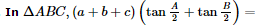Detailed Solution for Test: BITSAT Mock Test- 5 - Question 110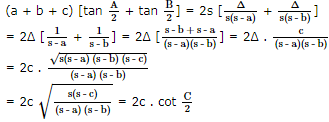Test: BITSAT Mock Test- 5 - Question 111
If b = 3, c = 4, and B = π/3, then the number of triangles that can be constructed is
Test: BITSAT Mock Test- 5 - Question 112

13 - 23 + 33 - 43 + ... + 93 =

Test: BITSAT Mock Test- 5 - Question 113

If one root of the equation x2 = px + q is reciprocal of the other and p≠±1. What is the value of q ?

Detailed Solution for Test: BITSAT Mock Test- 5 - Question 113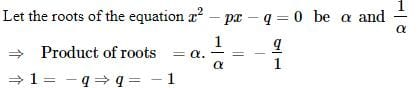Test: BITSAT Mock Test- 5 - Question 114

If A = [a,b], B = [c,d], C = [d,e] then {(a,c), (a,d),(a,e),(b,c),(b,d),(b,e)} is equal to

Test: BITSAT Mock Test- 5 - Question 115
The function f (x) = 10x from R to [0,∞] is
Test: BITSAT Mock Test- 5 - Question 116
If the first term of an A.P. is 2 and common difference is 4, then sum of its 40 terms is
Test: BITSAT Mock Test- 5 - Question 117

The equation of a line which makes an angle of 120o with x-axes and the length of perpendicular from origin on it is 4 units, is

Test: BITSAT Mock Test- 5 - Question 118
The equation of the normal to the curve y=sinx at (π,0) is
Test: BITSAT Mock Test- 5 - Question 119
The equation of the plane passing thro' the intersection of the planes x+2y+3z+4=0 and 4x+3y+2z+1=0 and the origin is
Test: BITSAT Mock Test- 5 - Question 120
The centre of sphere passing through four points (0, 0, 0), (0, 2, 0), (1, 0, 0) and (0, 0, 4) is
Test: BITSAT Mock Test- 5 - Question 121

If sin2x-2cosx+(1/4)=0, the general solution x is

Test: BITSAT Mock Test- 5 - Question 122

The values of θ satisfying sin 5θ = sin 3θ − sinθ and 0 < θ < π/2 are

Test: BITSAT Mock Test- 5 - Question 123

If x+y=a, x*y=b, x.a=1 then x and y is

Test: BITSAT Mock Test- 5 - Question 124

The value of cos 12o+cos 84o+cos 156o+cos 132o is

Test: BITSAT Mock Test- 5 - Question 125
The unit vector perpendicular to each of the vectors 2î-ĵ+k̂ and 3î+4ĵ is
Test: BITSAT Mock Test- 5 - Question 126
Find the Antonym of the word occurring in the sentence in capital letter as per the context.
Detailed Solution for Test: BITSAT Mock Test- 5 - Question 126 'Absurd' means not logical and sensible.
So its antonym will be Sensible
Test: BITSAT Mock Test- 5 - Question 127
Fill in the blank with appropriate word.
Hardly had he arrived .......... it started raining.
Test: BITSAT Mock Test- 5 - Question 128
Choose the one which can be substituted for the given words/sentences :
Thing that can be felt or touched
Test: BITSAT Mock Test- 5 - Question 129
Fill in the blank with appropriate word.
In a little publicised deal, Pepsi Cola has.......the entire soft drink market in Afghanistan.
Test: BITSAT Mock Test- 5 - Question 130
Find the Antonym of the word occurring in the sentence in capital letter as per the context.
His assignments have taken him to many EXOTIC locations around the world.
Detailed Solution for Test: BITSAT Mock Test- 5 - Question 130 'Exotic' means seeming exciting and unusual
Test: BITSAT Mock Test- 5 - Question 131
Choose the one which can be substituted for the given words/sentences
The custom of having more than one husband at the same time
Test: BITSAT Mock Test- 5 - Question 132
spot the error.
Test: BITSAT Mock Test- 5 - Question 133
Spot the error.
Test: BITSAT Mock Test- 5 - Question 134
Spot the error.
Detailed Solution for Test: BITSAT Mock Test- 5 - Question 134 Correct form is why he does not do the work
Test: BITSAT Mock Test- 5 - Question 135
Find the Synonym of the word occurring in the sentence in capital letter as per the context.
It is DEPLORABLE that millions of people in India are below the poverty line.
Detailed Solution for Test: BITSAT Mock Test- 5 - Question 135 'Deplorable' means bad and unacceptable
Test: BITSAT Mock Test- 5 - Question 136
Find the Synonym of the word occurring in the sentence in capital letter as per the context.
The students took COPIOUS notes during the lecture delivered by the visiting professor.
Detailed Solution for Test: BITSAT Mock Test- 5 - Question 136 'Copious' means abundant, plentiful.
In the given context, the question word means 'comprehensive'.
Hence the correct option is Exhaustive
Test: BITSAT Mock Test- 5 - Question 137
Choose the numeral pair which is different from others .
Test: BITSAT Mock Test- 5 - Question 138
Complete the analogous pair.
Fear : Threat : : Anger : ?
Test: BITSAT Mock Test- 5 - Question 139

Group the following figures into three classes on the basis of identical properties.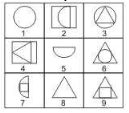Detailed Solution for Test: BITSAT Mock Test- 5 - Question 139

1,3,6 contain one complete circle each.
2,5,7 contain a semi-circle each.
4,8,9 contain a triangle each.

Test: BITSAT Mock Test- 5 - Question 140

Find the missing character.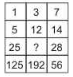Detailed Solution for Test: BITSAT Mock Test- 5 - Question 140

The sequence in first column is x 5. Thus, 1 x 5 = 5, 5 x 5 = 25, 25 x 5 = 125.
The sequence in third column is x 2. Thusx 7 x 2 = 14, 14 x 2 = 28, 28 x 2 = 56.
The sequence in second column is x 4.
∴ Missing number = 12 x 4 = 48.

Test: BITSAT Mock Test- 5 - Question 141
In this letter series, some of the letters are missing. Choose the correct letter given below -
_ acca _ ccca _ acccc _ aaa
Test: BITSAT Mock Test- 5 - Question 142
In this letter series, some of the letters are missing. Choose the correct letter given below -
_ aaba _ bba _ bba _ abaa _ b
Test: BITSAT Mock Test- 5 - Question 143

The martyrs who laid down their lives for the freedom of the country, had a lofty vision of the future. They wanted the nation to be free from all the slavery and bondage . They wanted an India in which all the communities would live in perfect harmony and in which there would be no high class and no low class of people, the curse of untouchability having been wiped out completely. Women would enjoy equal rights with men and contribute their fullest to the making of a great nation . Such a vision was in keeping with the ancient glory of the country renowned for its splendid achievements in literature , art and culture . We must now revitalise this ancient culture of ours with tolerance as its masthead. If we forget or cease to take pride, in our noble heritage , we shall have to face severe indictment in the court of history which is a ruthless judge and seldom spares the erring people.

Q. The martyrs who died for the freedom of India wanted:

Test: BITSAT Mock Test- 5 - Question 144

The martyrs who laid down their lives for the freedom of the country, had a lofty vision of the future. They wanted the nation to be free from all the slavery and bondage . They wanted an India in which all the communities would live in perfect harmony and in which there would be no high class and no low class of people, the curse of untouchability having been wiped out completely. Women would enjoy equal rights with men and contribute their fullest to the making of a great nation . Such a vision was in keeping with the ancient glory of the country renowned for its splendid achievements in literature , art and culture . We must now revitalise this ancient culture of ours with tolerance as its masthead. If we forget or cease to take pride, in our noble heritage , we shall have to face severe indictment in the court of history which is a ruthless judge and seldom spares the erring people.

Q. These martyrs wanted that :

Test: BITSAT Mock Test- 5 - Question 145

The martyrs who laid down their lives for the freedom of the country, had a lofty vision of the future. They wanted the nation to be free from all the slavery and bondage . They wanted an India in which all the communities would live in perfect harmony and in which there would be no high class and no low class of people, the curse of untouchability having been wiped out completely. Women would enjoy equal rights with men and contribute their fullest to the making of a great nation . Such a vision was in keeping with the ancient glory of the country renowned for its splendid achievements in literature , art and culture . We must now revitalise this ancient culture of ours with tolerance as its masthead. If we forget or cease to take pride, in our noble heritage , we shall have to face severe indictment in the court of history which is a ruthless judge and seldom spares the erring people.

Q. We must strive with our total commitment to :

Test: BITSAT Mock Test- 5 - Question 146

Q.